3.NF.1.Test7B1-2 Understand a fraction
 Name:    3.NF.1.Test7B1-2 Understand a fraction

Multiple Choice
Identify the choice that best completes the statement or answers the question.

1.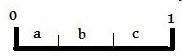Line 0 - 1 is a whole line.  What fraction of line 0 - 1 does section “a” represent?
 a. 1 c.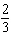b.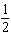d.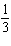2.Which letter can be found at 1/12 of the whole line?
 a. D c. A b. B d. E

3.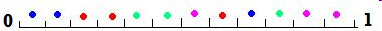What fraction of the whole line has blue and green colored dots?
 a.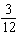c.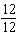b.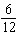d.4.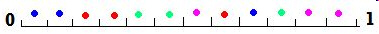What fraction of the whole line has colored dots?
 a.c.b.d.5.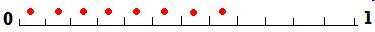What fraction of the whole line has red dots?
 a.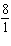c.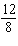b.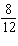d. 1

6.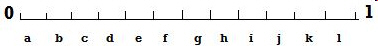What fraction of the whole line do letters e, f, g, h represent?
 a.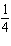c.b.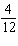d.7.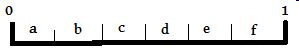Line 0 - 1 is a whole line.  What fraction of line 0 - 1 do sections “a, b, c, d,” represent?
 a.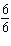c. 1 b.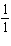d.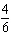8.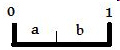Line 0 - 1 is a whole line.  What fraction of line 0 - 1 do sections “a and b” represent?
 a.c. 2 b.d. 1

9.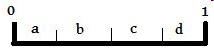Line 0 - 1 is a whole line.  What fraction of line 0 - 1 do sections “c and d” represent?
 a.c.b. 1 d. 2

10.Which letter can be found at 7/12 of the whole line?
 a. B c. E b. C d. A